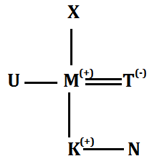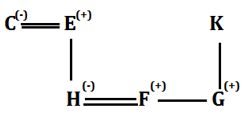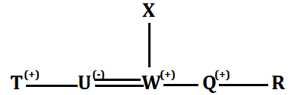Latest Banking jobs   »

# Reasoning Ability Quiz For SIDBI GRADE A 2023- 3rd January

Directions (1-3): In the following questions, the symbols #, &, @, *, \$, % and © are used with the following meanings as illustrated below. Study the following information and answer the given questions:
A@B- A is the child of B.
A©B- A is the parent of B
A%B- A is father-in-law of B
A&B- A is brother-in-law of B
A\$B- A is brother of B
A*B- A is wife of B
A#B- A is sister-in-law of B

Q1. If the expression ‘M©K\$N@T#U, X©M\$U’ is true, then which of the following is true?
(a) M is sister of U
(b) K is granddaughter of X
(c) T is mother of K
(d) N is child of U
(e) None of these

Q2. If the expression ‘C*E%F\$G@K, G&H@C’ is true, then who among the following is daughter-in-law of K?
(a) E
(b) H
(c) F
(d) C
(e) None of these

Q3. If the expression ‘T\$U*W@X©Q\$R’ is true, then who among the following is sister-in-law of R?
(a) W
(b) X
(c) T
(d) U
(e) None of these

Q4. The position of how many alphabets will remain unchanged if each of the alphabets in the word ‘QUANTITATIVE’ is arranged in alphabetical order from left to right?
(a) Four
(b) One
(c) Two
(d) Three
(e) None

Q5. How many pairs of letters are there in the word “COUNSELING” each of which have as many letters between them in the word as they have between them in the English alphabetical series?
(a) Four
(b) One
(c) Two
(d) Three
(e) More than four

Directions (6-10): In the following questions assuming the given statement to be true, find which of the conclusion(s) among given conclusions is/are definitely true and then give your answers accordingly.

Q6. Statements: Q > A = E ≥ S = T, J = A > L ≥ M, Y = R ≥ A > O
Conclusions: I. Y < Q
II. Y > M
(a) Only conclusion I is true
(b) Only conclusion II is true
(c) Either conclusion I or II is true
(d) Both conclusions I and II are true
(e) Neither conclusion I nor II is true

Q7. Statements: G < Q = C ≤ N = X, S > N = E > M, Z = J = S > L
Conclusions: I. Z > G
II. L > C
(a) Only conclusion I is true
(b) Only conclusion II is true
(c) Either conclusion I or II is true
(d) Both conclusions I and II are true
(e) Neither conclusion I nor II is true

Q8. Statements: N ≤ T = H < M, N = W ≥ K > S, P ≤ M = D
Conclusions: I. H > S
II. W < M
(a) Only conclusion I is true
(b) Only conclusion II is true
(c) Either conclusion I or II is true
(d) Both conclusions I and II are true
(e) Neither conclusion I nor II is true

Q9. Statements: V ≤ P = C ≤ H, N ≥ C < E = Y, P = L ≤ S = T
Conclusions: I. L > Y
II. N ≥ V
(a) Only conclusion I is true
(b) Only conclusion II is true
(c) Either conclusion I or II is true
(d) Both conclusions I and II are true
(e) Neither conclusion I nor II is true

Q10. Statements: I = E > W < L, D ≤ F = E < X, X < T = G > A
Conclusions: I. I < G
II. L < A
(a) Only conclusion I is true
(b) Only conclusion II is true
(c) Either conclusion I or II is true
(d) Both conclusions I and II are true
(e) Neither conclusion I nor II is true

Directions (11-13): In the following questions assuming the given statement to be true, find which of the conclusion(s) among given conclusions is/are definitely true and then give your answers accordingly.

Q11. Statements: G > I ≥ C, C = D > E, G ≥ F
Conclusions: I. G > C
II.F > I
(a) Only I is true
(b) Only II is true
(c) Either I or II is true
(d) Neither I nor II is true
(e) Both I and II are true

Q12. Statements: G > I ≥ C, C = D > E, G ≥ F
Conclusions: I. G > D
II.G = D
(a) Only I is true
(b) Only II is true
(c) Either I or II is true
(d) Neither I nor II is true
(e) Both I and II are true

Q13. Statements: G > I ≥ C, C = D > E, G ≥ F
Conclusions: I. F > C
II. F ≤ C
(a) Only I is true
(b) Only II is true
(c) Either I or II is true
(d) Neither I nor II is true
(e) Both I and II are true

Directions (14-15): In the following questions, a statement(s) are given followed by some conclusions. Study the following data carefully and answer the questions accordingly.

Q14. Statements: U = X ≥ I, C ≤ D > I = L ≥ G > H
Conclusions: I. G ≤ I
II. L < U
(a) If only conclusion I follows
(b) If only conclusion II follows
(c) If either conclusion I or II follows
(d) If neither conclusion I nor II follows
(e) If both conclusions I and II follow

Q15. Statements: A > J = K ≥ Z = M ≤ O, J < R ≤ V
Conclusions: I. Z < O
II. O = Z
(a) If only conclusion I follows
(b) If only conclusion II follows
(c) If either conclusion I or II follows
(d) If neither conclusion I nor II follows
(e) If both conclusions I and II follow

Solutions

S1. Ans. (c)
Sol.S2. Ans. (b)
Sol.S3. Ans. (d)
Sol.S4. Ans. (b)

S5. Ans. (e)

S6. Ans. (b)
Sol. I. Y < Q – False
II. Y > M – True

S7. Ans. (a)
Sol. I. Z > G – True.
II. L > C – False

S8. Ans. (d)
Sol. I. H > S – True.
II. W < M – True.

S9. Ans. (b)
Sol. I. L > Y – False
II. N ≥ V – True.

S10. Ans. (a)
Sol. I. I < G – True.
II. L < A – False

S11. Ans. (a)
Sol. I. G > C – True.
II.F > I – False

S12. Ans. (a)
Sol. I. G > D – True.
II.G = D – False

S13. Ans. (c)
Sol. I. F > C – False
II. F ≤ C – False

S14. Ans. (a)
Sol. I. G ≤ I – True.
II. L < U – False

S15. Ans. (c)
Sol. I. Z < O – False
II. O = Z – False#### Congratulations!